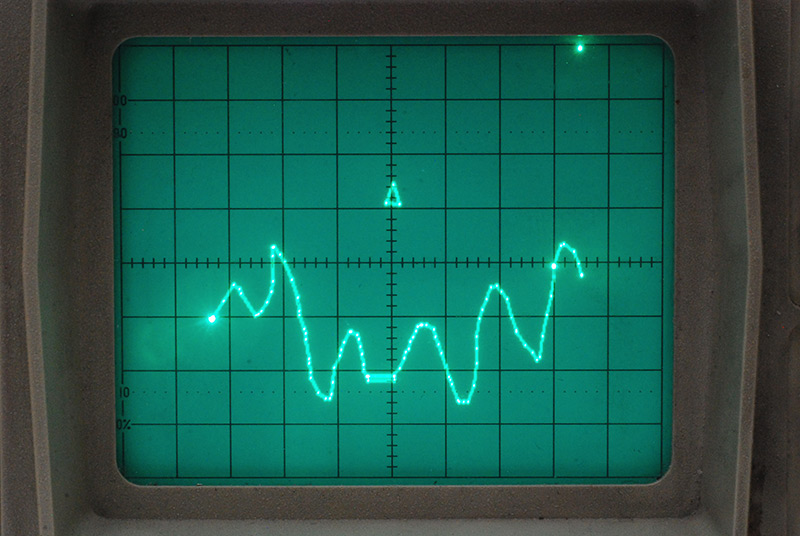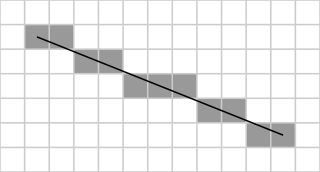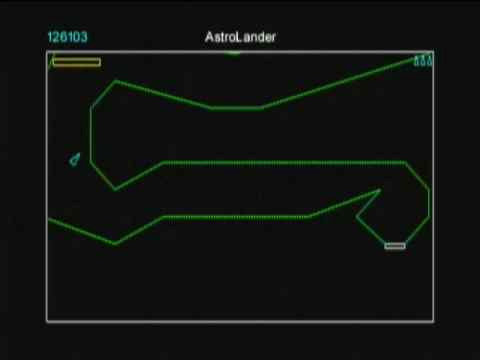# Astrolander

22 Dec 2018
Progress: Complete

This is page 8 of the Games Console project.

I wanted to complete the trilogy, but part of the way through this one I got distracted and started working on the next game. But, there are still a few interesting points to note here.### Lines

In bitmap graphics, Bresenham's line algorithm is used to draw a straight line between two points.If you've been looking through the source code so far, you may have been surprised to see the implementation of Bresenham's line algorithm here. Certainly my first thought about vector graphics was that we wouldn't need to worry about this sort of thing, but no, it's actually really important.

Slew rate is the speed at which an output voltage can change. If we tell our video circuit to output a certain value, it will always take a finite amount of time to reach that point. In fact, it'll approach the value exponentially. This is an important point. If we tried to draw a straight line between two points on the display by simply oscillating between the end points, the beam would move exponentially from point to point, and so for anything except 90 or 45 degree angles, instead of a line, we'd end up with a lemon-shape, as the X and Y movements travelled at different speeds.

So we need to use the line algorithm and move a pixel at a time, if we want our lines to be straight. Luckily, it's quite straight forward and I just copied the logic from wikipedia. The real question is, why do old vector games like Asteroids not look pixellated? Well, they are, but they run at a higher resolution so you don't notice it. The real benefit of vector graphics is from the days of limited video memory. With vector graphics, you can have a higher resolution for a given amount of video memory. In our case, however, we were rather silly and went for a low resolution vector output. And also, we have no video memory at all. 90% of our CPU time is spent in the drawing routines.

There is a subtle edge-case in our implementation of the line algorithm. The screen coordinates are all unsigned, but the algorithm works with signed offsets. In order for lines longer than 128 pixels to be drawn correctly, we have to do some extra checking at the start of the routine.

For Astrolander, the example map is something I quickly sketched out in paint, and the points are so close together you that may think the line algorithm isn't needed, but it's all the more important when we start to zoom in on the scene. Also, the real maps, if I ever got as far as ripping them, were mostly composed of straight lines.### Scaling

Acceleration and momentum are pretty much just extensions of what we did with the RetroRacer movement.

Scaling means we can't get away with limited resolution, everything needs to be at least 16-bit. With a 16-bit scale factor and 16-bit coordinates, applying the scale means double precision multiplications. Extending the precision of the multiply instructions is a fairly standard proceedure, I think there are even examples of it in the datasheets. It's pretty much a case of high byte times low byte, low byte times high byte, high times high, low times low, and add them all in the right proportions.

```; Function scaleCoordinate - modified 16bit signed multiply.
; r21:r20 = scale * r21:r20
scaleCoordinate:
push r16
push r17
push r18
push r19
clr r2
muls scaleH, r21 ; (signed)ah * (signed)bh
movw r19:r18, r1:r0
mul scaleL, r20 ; al * bl
movw r17:r16, r1:r0
mulsu scaleH, r20 ; (signed)ah * bl
sbc r19, r2
mulsu r21, scaleL ; (signed)bh * al
sbc r19, r2
sbrs r19,7 ; if result is negative, don't round
inc r2
sbrc r17,7 ; round to 16bit result
clr r2
movw r21:r20, r19:r18
pop r19
pop r18
pop r17
pop r16
ret
```

Once we've scaled our coordinate, we then need to only draw it if it's within the display. A modified drawLine routine checks for this, and attempts to draw only part of the line if it's half-in half-out.

I didn't write these games in the order they're presented here, or in the video. I think AstroLander was actually the penultimate game I made, right before mario. Looking through the source code, the style is quite different to how I was writing things at the beginning.

If we'd gotten as far as it, collisions would have been an interesting challenge. We'd really want this to be pixel accurate, so I suppose the only viable way would be to perform a set of comparisons within the line-drawing routines for the terrain. Each pixel in the bresenham implementation would have to be checked against the stack of pixels that compose the triangle that is our spaceship. I'm not sure if we'd have enough processing power for this, but it could probably be inserted in place of the delay routines as it's drawn. As the triangle of the spaceship got bigger, there would be less terrain to check against. Possibly, if needed, a pre-comparison could check if the spaceship is nearby or miles away, and skip the bulk of the code if it isn't needed.

Not much more to be said about this one. Dig in to the juicy, half-finished code below. Commented out is an unfinished auto-scaling routine, but otherwise the zoom is just set by pressing up and down on the controller. Next up, Tetris and the 3D malarky.

```### AstroLander.asm ###

;init frame counter
ldi r16,0
out TCNT0, r16
ldi r16, (1<<CS02)|(1<<CS01)|(1<<CS00)
out TCCR0, r16
ldi r16, 128
out OCR0, r16

.def x_H = r4
.def x_L = r5
.def y_H = r6
.def y_L = r7
.def speedx_H = r8
.def speedx_L = r9
.def speedy_H = r10
.def speedy_L = r11
.def theta = r12

.def translateX = r14
.def translateY = r15
.def scaleH = r22
.def scaleL = r23

ldi scaleH,2    ;2.5
ldi scaleL,128
ldi r16,128
mov translateX,r16
ldi r16,220
mov translateY,r16

ldi r16,0
mov speedx_H,r16
mov speedx_L,r16
mov speedy_H,r16
mov speedy_L,r16
mov x_H,r16
mov y_H,r16

Main:

;////////

sbrs r2,ctrlUp
rjmp asdf
subi scaleL,-1
sbci scaleH,-1
asdf:
sbrs r2,ctrlDown
rjmp fdsa
subi scaleL,1
sbci scaleH,0
fdsa:

;/////////

ldi r16, 2 ; turn speed
sbrc r2, ctrlLeft
sbrc r2, ctrlRight
sub theta,r16

; Accelerate
sbrs r2, ctrlA
rjmp doGravity

mov r17, theta
ldi r16, 16                 ; acceleration magnitude
rcall sinS                  ; returns in r1:r0
sbrc r0,7
inc r1
ldi r16,0
sbrc r1,7
ldi r16,-1

mov r17, theta
ldi r16, 16                 ; acceleration magnitude
rcall cosS                  ; returns in r1:r0
sbrc r0,7
inc r1
ldi r16,0
sbrc r1,7
ldi r16,-1

doGravity:

ldi r16,4
ldi r16,0

rcall friction

;push r2

/*
; calculate scaling
rcall calcDistance
ldi r16,10
cp r1,r16
brcc notclose
;lsl scaleL
;rol scaleH
subi scaleL,-8
sbci scaleH,-1
rjmp donescaling
notclose:
ldi r16,1
ldi r17,0
cp scaleL,r17
cpc scaleH,r16
brcs donescaling
;lsr scaleH
;ror scaleL
subi scaleL,8
sbci scaleH,0

donescaling:
*/
/*
ldi ZH, HIGH(scalingLookup*2)
ldi ZH,  LOW(scalingLookup*2)
clr r0
lpm scaleL, Z+
lpm scaleH, Z+
*/

;pop r2
rcall drawShip

; draw map
/*
ldi r16,-10
ldi r17,-5
ldi r18,4
ldi r19,-12
ldi r26,0
ldi r27,0
ldi r24,0
ldi r25,0
rcall drawLineScaled
*/
ldi ZH, HIGH(polygon1*2)
ldi ZL,  LOW(polygon1*2)
rcall drawPolygonPM
ldi ZH, HIGH(polygon2*2)
ldi ZL,  LOW(polygon2*2)
rcall drawPolygonPM

ldi r16,0
out PORTA,r16
out PORTC,r16
waitforframe:
in r16, TIFR
sbrs r16, OCF0
rjmp waitforframe
ldi r16, (1<<OCF0)
out TIFR, r16
ldi r16, 0
out TCNT0, r16
rjmp Main

drawShip:
push r2

ldi r16,8
mov r17, theta
rcall sinS
movw r30, r0        ; r31:r30 is now sintheta
ldi r16,8
mov r17, theta
rcall cosS
movw r28, r0        ; r29:r28 is now costheta

; r16 = x0H
; r17 = y0H
; r18 = x1H
; r19 = y1H

; r26 = x0L
; r27 = y0L
; r24 = x1L
; r25 = y1L

mov r26,x_L
mov r16,x_H
add r26,r28 ; x + costheta

mov r27,y_L
mov r17,y_H
add r27,r30 ; y + sintheta

push r16    ; save for later
push r17
push r26
push r27

asr r31     ; Halve sin/cos values
ror r30
asr r29
ror r28

mov r24,x_L
mov r18,x_H
add r24,r30 ; x + halfsin - halfcos
sub r24,r28
sbc r18,r29

mov r25,y_L
mov r19,y_H
sub r25,r30 ; y -halfcos-halfsin
sbc r19,r31
sub r25,r28
sbc r19,r29

rcall drawLineScaled

mov r24,x_L
mov r18,x_H
sub r24,r30 ; x - halfsin - halfcos
sbc r18,r31
sub r24,r28
sbc r18,r29

mov r25,y_L
mov r19,y_H
sub r25,r30 ; y +halfcos-halfsin
sbc r19,r31

rcall drawLineScaled

pop r25     ; recover starting position
pop r24
pop r19
pop r18

rcall drawLineScaled

pop r2
sbrs r2,ctrlA
rjmp drawShipEnd    ; draw the plume

mov r26,x_L
mov r16,x_H
add r27,r30 ; x + halfsin - halfcos
sub r27,r28
sbc r16,r29

mov r27,y_L
mov r17,y_H
sub r28,r30 ; y -halfcos-halfsin
sbc r17,r31
sub r28,r28
sbc r17,r29

mov r24,x_L ; x-costheta
mov r18,x_H
sub r24,r28
sbc r18,r29
sub r24,r28
sbc r18,r29

mov r25,y_L
mov r19,y_H
sub r25,r30 ; y -sintheta
sbc r19,r31
sub r25,r30
sbc r19,r31

rcall drawLineScaled

mov r24,x_L
mov r18,x_H
sub r24,r30 ; x - halfsin - halfcos
sbc r18,r31
sub r24,r28
sbc r18,r29

mov r25,y_L
mov r19,y_H
sub r25,r30 ; y +halfcos-halfsin
sbc r19,r31

rcall drawLineScaled

drawShipEnd:
ret

; Function friction
; subtracts one sixteenth of current speed from speed
friction:
push r16
push r17
clr r17
sbrc speedx_H,7
dec r17
mov r16, speedx_H
sub speedx_L, r16
sbc speedx_H,r17
clr r17
sbrc speedy_H,7
dec r17
mov r16, speedy_H
sub speedy_L, r16
sbc speedy_H,r17
pop r17
pop r16
ret

; function calcDistance
; Works out the squared distance between x_H,y_H and 0,0
calcDistance:
;ldi r16,0
;ldi r17,0
mov r26,x_H
;sub r26,r16        ; Difference between x coordinates
muls r26,r26    ; square it
movw r24,r0     ; save for later
mov r26,y_H
;sub r26,r17        ; difference in y coordinates
muls r26,r26    ; square it
adc r1,r25      ; (return in r1:r0)

ret

; Function scaleCoordinate - modified 16bit signed multiply.
; r21:r20 = scale * r21:r20
scaleCoordinate:
push r16
push r17
push r18
push r19
clr r2
muls scaleH, r21 ; (signed)ah * (signed)bh
movw r19:r18, r1:r0
mul scaleL, r20 ; al * bl
movw r17:r16, r1:r0
mulsu scaleH, r20 ; (signed)ah * bl
sbc r19, r2
mulsu r21, scaleL ; (signed)bh * al
sbc r19, r2
sbrs r19,7 ; if result is negative, don't round
inc r2
sbrc r17,7 ; round to 16bit result
clr r2
movw r21:r20, r19:r18
pop r19
pop r18
pop r17
pop r16
ret

/*
; Transform functions - scale and translate.
transformX:
mov r21,x_H
mov r20,x_L
rcall scaleCoordinate
ret

transformY:
mov r21,y_H
mov r20,y_L
rcall scaleCoordinate
ret
*/

/* Function drawPolygonPM(Z pointer)
* Draw from a list of coords in program memory
* First byte is number of vertices, followed by pairs of x,y bytes
*/
drawPolygonPM:

.def vertexCount=r3

lpm vertexCount, Z+
lpm r16,Z+              ; load starting point
lpm r17,Z+              ; all polygons must have at least 2 vertices
dec vertexCount

polyPMnextVert:
lpm r18,Z+              ; load next vertex
lpm r19,Z+
ldi r26,0
ldi r27,0
ldi r24,0
ldi r25,0

rcall drawLineScaled

dec vertexCount
brne polyPMnextVert     ; repeat until end of polygon

ret

drawLineScaled:
; Transform start and end coordinates.
; If within the screen, call ordinary drawLine.
; r16 = x0H
; r17 = y0H
; r18 = x1H
; r19 = y1H

; r26 = x0L
; r27 = y0L
; r24 = x1L
; r25 = y1L

push r18    ; save final coordinate
push r19
push r24
push r25

; scale x0
mov r21,r16
mov r20,r26
rcall scaleCoordinate
cpi r21, 0
breq dLx0WithinRange

; x0 is out of range
rjmp scaleLine

dLx0WithinRange:
push r20    ; x0 ok

; scale y0
mov r21, r17
mov r20, r27
rcall scaleCoordinate
cpi r21,0
breq dLy0WithinRange

; y0 is out of range
pop r20
rjmp scaleLine

dLy0WithinRange:
push r20

; scale x1
mov r21,r18
mov r20,r24
rcall scaleCoordinate
cpi r21, 0
breq dLx1WithinRange

; x1 is out of range
pop r20
pop r20
rjmp scaleLine

dLx1WithinRange:
push r20

; scale y1
mov r21, r19
mov r20, r25
rcall scaleCoordinate
cpi r21,0
breq dLy1WithinRange

; y1 is out of range
pop r20
pop r20
pop r20
rjmp scaleLine

dLy1WithinRange:
mov r19,r20
pop r18
pop r17
pop r16

rcall drawLine

scaleLine:
; for now, just don't draw it.

pop r27     ;shift end point to starting point (for polygons)
pop r26
pop r17
pop r16

ret

/*
;Local variables
push r20
push r21
push r22
push r23
push r24
push r25
.def slowx=r20
.def slowy=r21
.def fastx=r22
.def fasty=r23
.def err = r24
.def t   = r25

; First we split the magnitude and direction
; Need to be careful/explicit because mag could be >127

cp dx,x0
breq slineXeq
brcs slineXneg      ; Carry flag set if it overflowed

ldi slowx,1         ; slowx is the direction
sub dx,x0           ; dx is now the magnitude
rjmp slineY

slineXneg:
ldi slowx,-1
mov t, x0           ; t as temp var
sub t, dx
mov dx, t
rjmp slineY

slineXeq:
clr slowx
clr dx

slineY:                 ; Now do the same for y
cp dy,y0
breq slineYeq
brcs slineYneg

ldi slowy,1
sub dy,y0
rjmp slineCheckDistance

slineYneg:
ldi slowy,-1
mov t, y0           ; t as temp var
sub t, dy
mov dy, t
rjmp slineCheckDistance

slineYeq:
clr slowy
clr dy

slineCheckDistance:     ; Now to find which direction is "faster"
cp dx, dy
brcs slineDxLessthanDy

mov fastx, slowx    ; x is the fast direction
clr fasty

eor dx, dy          ; Tripple XOR, the classy way to
eor dy, dx          ; swap two registers
eor dx, dy

rjmp slineInit

slineDxLessthanDy:
mov fasty, slowy    ; y is the fast direction, no need to swap
clr fastx

slineInit:
mov err, dy         ; set err to half the furthest distance
lsr err

out PORTA, x0       ; We are now ready to draw the line
out PORTC, y0
rcall delayPixel

mov t, dy

slineNextPixel:
sub err, dx         ; Update error term
brcc slineStepParallel

add err, dy         ; Make error term positive again

rjmp slineLoopBack

slineStepParallel:

slineLoopBack:
out PORTA, x0       ; Plot point
out PORTC, y0
rcall delayPixel

dec t
brne slineNextPixel

pop r25
pop r24
pop r23
pop r22
pop r21
pop r20

ret                 ; End of drawLine

*/

/*  Function drawLine (x0,y0,x1,y1)
*  My implementation of Bresenham line algorithm
*  based on pseudocode/description from wikipedia
*/
drawLine:

;Function arguments
.def x0=r16
.def y0=r17
.def dx=r18
.def dy=r19

;Local variables
push r20
push r21
push r22
push r23
push r24
push r25
.def slowx=r20
.def slowy=r21
.def fastx=r22
.def fasty=r23
.def err = r24
.def t   = r25

; First we split the magnitude and direction
; Need to be careful/explicit because mag could be >127

cp dx,x0
breq lineXeq
brcs lineXneg       ; Carry flag set if it overflowed

ldi slowx,1         ; slowx is the direction
sub dx,x0           ; dx is now the magnitude
rjmp lineY

lineXneg:
ldi slowx,-1
mov t, x0           ; t as temp var
sub t, dx
mov dx, t
rjmp lineY

lineXeq:
clr slowx
clr dx

lineY:                  ; Now do the same for y
cp dy,y0
breq lineYeq
brcs lineYneg

ldi slowy,1
sub dy,y0
rjmp lineCheckDistance

lineYneg:
ldi slowy,-1
mov t, y0           ; t as temp var
sub t, dy
mov dy, t
rjmp lineCheckDistance

lineYeq:
clr slowy
clr dy

lineCheckDistance:      ; Now to find which direction is "faster"
cp dx, dy
brcs lineDxLessthanDy

mov fastx, slowx    ; x is the fast direction
clr fasty

eor dx, dy          ; Tripple XOR, the classy way to
eor dy, dx          ; swap two registers
eor dx, dy

rjmp lineInit

lineDxLessthanDy:
mov fasty, slowy    ; y is the fast direction, no need to swap
clr fastx

lineInit:
mov err, dy         ; set err to half the furthest distance
lsr err

out PORTA, x0       ; We are now ready to draw the line
out PORTC, y0
rcall delayPixel

mov t, dy

lineNextPixel:
sub err, dx         ; Update error term
brcc lineStepParallel

add err, dy         ; Make error term positive again

rjmp lineLoopBack

lineStepParallel:

lineLoopBack:
out PORTA, x0       ; Plot point
out PORTC, y0
rcall delayPixel

dec t
brne lineNextPixel

pop r25
pop r24
pop r23
pop r22
pop r21
pop r20

ret                 ; End of drawLine

delayPixel:
push r16
ldi r16,12
delayPixelLoop:
dec r16
brne delayPixelLoop
pop r16
ret

/* Function sinS(coordinate, dir)
* Scales 8bit signed coordinate by sine of the direction
* Simplified, no rounding, returns in r1:r0
*/
sinS:
.def cor=r16
.def dir=r17
push ZH
push ZL
ldi ZH, HIGH(sineTable*2+255)
ldi ZL,  LOW(sineTable*2+255)
sub ZL, dir
sbci ZH, \$00            ; sub-with-carry 0 from high byte
lpm dir, Z
fmuls cor,dir
pop ZL
pop ZH
ret

/* Function cosS(coordinate, dir)
* Scales 8bit signed coordinate by cosine of the direction
*/
cosS:
push ZH
push ZL
ldi ZH, HIGH(sineTable*2+255)
ldi ZL,  LOW(sineTable*2+255)   ; reuse table, but shift by pi/2
subi dir, 192                   ; which in our notation is 64 (-192)
sub ZL, dir
sbci ZH, \$00
lpm dir, Z
fmuls cor,dir
pop ZL
pop ZH
ret

sbis SPSR,SPIF
rjmp readController     ; Wait for reception complete
com r2

push r16
ldi r16,0b00000000      ; init controller read again
out SPDR,r16
pop r16
ret

; signed values, in reverse order
sineTable:

polygon2:
.db 63,  0,0,-3,-3,-6,-10,-7,-13,-9,-15,-10,-20,-11,-22,-13,-26,-14,-29,-16,-30,-17,-30,-20,-30,-24,-27,-25,-22,-27,-18,-29,-12,-31,-4,-33,2,-34,4,-35,6,-39,16,-40,17,-42,17,-45,17,-49,7,-51,-6,-51,-15,-51,-22,-52,-33,-54,-39,-57,-45,-60,-52,-63,-53,-66,-50,-70,-45,-72,-34,-75,-26,-75,-25,-81,-17,-85,-12,-88,-8,-90,-10,-92,-22,-94,-35,-97,-47,-99,-59,-99,-65,-99,-65,-99,-66,-103,-78,-105,-79,-112,-74,-114,-67,-116,-60,-119,-48,-120,-38,-121,-29,-121,-17,-123,-11,-124,-8,-125,-7,-126,-4,-128,-3

polygon1:
.db 55,  0,1,16,1,16,4,0,4,0,1,17,1,19,-2,19,-6,20,-12,21,-17,22,-21,23,-24,26,-26,27,-26,31,-20,33,-15,34,-11,37,-4,38,5,39,12,40,15,43,15,47,11,52,2,52,-1,53,-7,53,-10,55,-21,55,-25,56,-28,58,-36,58,-39,59,-47,63,-58,67,-69,70,-73,74,-78,75,-78,75,-72,75,-57,76,-51,78,-44,82,-39,84,-37,86,-36,95,-50,96,-52,99,-55,106,-45,113,-34,113,-34,113,-34,122,-33,125,-36,127,-41